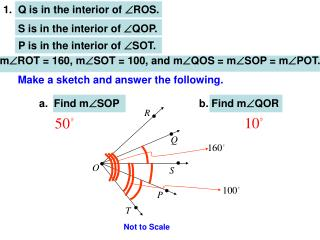DownloadDownload PresentationS is in the interior of  QOP.

# S is in the interior of  QOP.

Download Presentation## S is in the interior of  QOP.

- - - - - - - - - - - - - - - - - - - - - - - - - - - E N D - - - - - - - - - - - - - - - - - - - - - - - - - - -
##### Presentation Transcript

1. 1. Q is in the interior of ROS. S is in the interior of QOP. P is in the interior of SOT. mROT = 160, mSOT = 100, and mQOS = mSOP = mPOT. Make a sketch and answer the following. a. Find mSOP b. Find mQOR Not to Scale

2. 2. Translate the statement to “if→then” form. A beagle is always a reminder of Snoopy. If the dog is a beagle, then it reminds me of snoopy. Snoopy Beagle Could other things remind me of snoopy? Yes!

3. State the hypothesis and conclusion of the conditional, • then write the statement’s converse. If you flip the switch, then the light will come on.

4. Describe a counter-example that could demonstrate thegiven statement is false. If you cheat on the exam, then you will pass. I cheated on the exam and did not pass because…!

5. 5.Write the statement in biconditional form “if and only if” The sum of the measures of complementary angles is 90 degrees.

6. 6. Complete the argument by filling the missing portion of each conditional statement using the surrounding statements. Theorem: If the moon were made of green cheese, then it would be one giant peep for mousekind. If the moon were made of green cheese, ___________________________________. If mice were eager astronauts, sooner or later NASA would send some on a lunar mission. ________________________________, the eyes of the entire world would be watching them on television. _______________________________________, it would be one giant peep for mouse kind.

7. In each of the following proofs, provide the reason that justifies each statement in the left column. May be anything we have learned to date. 7. Given: x + 3 = 7 – x Prove: x = 2 Statement Reason 1. x + 3 = 7 – x 2. 2x + 3 = 7 3. 2x = 4 4. x = 2 1. 2. 3. 4. QED

8. 8. Given: AB = CD Prove: AC = BD C D B A Statement Reason 1. AB = CD 2. BC = BC 3. AB + BC = CD + BC 4. AB + BC = AC 5. BC + CD = BD 6. AC = BD 1. 2. 3. 4. 5. 6. QED

9. S R 9. Given: PR bisects SPQ Prove: 2(mRPQ) = mSPQ P Q Statement Reason 1. PR bisects SPQ 2. mRPQ = mRPS 3. mRPQ + mRPS = mSPQ 4. mRPQ + mRPQ = mSPQ 5. 2(mRPQ) = mSPQ 1. 2. 3. 4. 5. QED